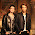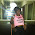## Saturday, July 23, 2011

### External Sorting for sorting large files in disk

Sorting is a fundamental programming task. Given the abundance of built-in libraries that perform tasks like sorting and binary search, we often become forgetful of exactly how these tasks are accomplished.

When the data is so large that it cannot be processed in memory at one time we need to resort to the file system to store part or all the data during the sorting process. We then need to perform another layer of disk operations on top of regular sorting algorithms to manage the data as they get sorted.

External Sorting is precisely the technique we described in the previous paragraph.

Let us describe in some detail how external sorting can be done in Java:

First the algorithm:

Say, we have one file (it can be more than one file, but having just one file simplifies the process for illustration purpose) in disk containing N numbers. And suppose the memory in our computer can hold M numbers at a time.

1. Start reading the input file from the beginning.
2. Read M (or less if number of entries remaining in the file is less than M) numbers from the file and store it into a temp buffer.
3. Sort (using any good sorting method - Quicksort, for example) the numbers in the buffer stored in step 2.
4. Create a temp file in disk and write out the sorted numbers from step 3 to this temp file. Save the name of the temp file.
5. Repeat step 2 to 4 until all numbers from the input file has been read, sorted, and written out to temp files.

At this point, we have chunks of numbers of size M sorted and stored in temp files in disk. We need to merge all these sorted files into one single sorted file. We will apply the merging algorithm from Merge Sort to join the numbers from these sorted files together.

6. Open all the temp files (and set the read pointer to the beginning of the files).
7. Find the minimum number from the set of numbers currently pointed to by the file read pointer.
8. Write the number to disk. (To increase efficiency you could write the number to a buffer first and then flush the buffer out to disk when the buffer is full. But modern I/O libraries should be doing this anyway for you).
9. Read another number from the file that contained the minimum number at step 7.
10. Repeat step 7 to 9 until all numbers from all the temp files have been processed, merged, and written out to disk.

The new file in disk now contains a sorted list of the numbers supplied in the initial input file.

Java code:

import java.io.FileNotFoundException;
import java.io.FileWriter;
import java.io.IOException;
import java.io.PrintWriter;
import java.util.Arrays;
import java.util.Random;

public class ExternalSort
{
static int N = 2000000; // size of the file in disk
static int M = 100000; // max items the memory buffer can hold

public static void externalSort(String fileName)
{
String tfile = "temp-file-";
int[] buffer = new int[M < N ? M : N];

try
{
int slices = (int) Math.ceil((double) N/M);

int i, j;
i = j = 0;
// Iterate through the elements in the file
for (i = 0; i < slices; i++)
{
// Read M-element chunk at a time from the file
for (j = 0; j < (M < N ? M : N); j++)
{
if (t != null)
buffer[j] = Integer.parseInt(t);
else
break;
}
// Sort M elements
Arrays.sort(buffer);

// Write the sorted numbers to temp file
FileWriter fw = new FileWriter(tfile + Integer.toString(i) + ".txt");
PrintWriter pw = new PrintWriter(fw);
for (int k = 0; k < j; k++)
pw.println(buffer[k]);

pw.close();
fw.close();
}

br.close();
fr.close();

// Now open each file and merge them, then write back to disk
int[] topNums = new int[slices];

for (i = 0; i < slices; i++)
{
if (t != null)
topNums[i] = Integer.parseInt(t);
else
topNums[i] = Integer.MAX_VALUE;
}

FileWriter fw = new FileWriter("E:\\test\\external-sorted.txt");
PrintWriter pw = new PrintWriter(fw);

for (i = 0; i < N; i++)
{
int min = topNums;
int minFile = 0;

for (j = 0; j < slices; j++)
{
if (min > topNums[j])
{
min = topNums[j];
minFile = j;
}
}

pw.println(min);
if (t != null)
topNums[minFile] = Integer.parseInt(t);
else
topNums[minFile] = Integer.MAX_VALUE;

}
for (i = 0; i < slices; i++)
brs[i].close();

pw.close();
fw.close();
}
catch (FileNotFoundException e)
{
e.printStackTrace();
} catch (IOException e) {
e.printStackTrace();
}
}

static String generateInput(int n)
{
String fileName = "external-sort.txt";
Random rand = new Random();

try
{
FileWriter fw = new FileWriter(fileName);
PrintWriter pw = new PrintWriter(fw);

for (int i = 0; i < n; i++)
pw.println(rand.nextInt(101));

pw.close();
}

catch (IOException e)
{
e.printStackTrace();
}

return fileName;
}

public static void main(String[] args)
{
String fileName = generateInput(N);
externalSort(fileName);
}
}

Happy external sorting!

1.Good one Bilash Bhaia!

Rajib

2.Thanks Rajib:)

3.Hi, Golam Kawsar

I checked your code. It opened a lot of temp file .txt. Do you know how to close these files by putting some code in your program to close these file?

It creates 22 file txt. 1 file for external sort, 1 file of external sorted, and the rest are temp files. Inside these temp files, they just same exactly others, adn same like external sorted file.

4.By close do you mean deleting the files? You can delete the files using the delete() function in the File class.

5.I tried but it not work, maybe I put it in wrong location. Can you show me specific where to delete, and the code to delete those temp-file, after it done its work.

Thank you

6.When you look for the minimum element, you go through all the smallest numbers of all the slices every time, which is a O(n) operation, where n = size of each slice.
You can rather use a minHeap to maintain the smallest number at the top at all times, that would be O(log(n)) operation.

1.Hi Arpita,

7.Yeah that's good idea. The Heap will act as sort of a priority queue where you can pop the min element with O(1) time.

8.This comment has been removed by the author.

9.Good post!

I have just built some abstract structures called big queue and big array which can be used to sort and search big data in a simple and elegant way. Basically, the algorithm used is similar to yours - external merge sort.

I can sort 128GB data(100 bytes each data item) in 9 hours on a single machine, and then binary search the sorted data with almost no time.

Here is a post about how to search big data by using the big queue and big array structures:
http://bulldog2011.github.com/blog/2013/01/25/merge-sort-using-big-queue/

10.Great work William. Thanks for reading my post and leaving a comment.

11.Nice post !

I have a quick question: say you have a 10GB file (one number per line) and you have 100MB memory to use. what's the size for the buffer then? How do you know how many lines to read before reading lines for the next file?

12.Hi,

How do we sort 40Gb data when you have 16Gb mem and only 40Gb diskspace left.

Is it possible to sort?

13.If we only have 40GB disk space (all of which is used to for the input numbers) and only 16GB memory, we can still apply the algorithm described here. But this will be a bit more involved and will require a lot of file pointer manipulation so that we can use the 40GB disk space for the sorted temp files produced by the algorithm.

14.Thank you so much for providing this algorithm! Very clean and works nicely.

15.Great work done...can u please tell what the input for this code should be? I know the question is trivial but pls answer

1.Hi, thanks for reading the post. The program does not accept any external input. It produces the data (in file) using the provided generateInput() method and then sorts those numbers.

2.Hi , I tried executing the code but it threw few error which i'm not able to correct.Could you pls help me with this ?

16.Can u pls provide me with ur Email ID so that i can contact you regarding this.I'm actually in need of this code.Thanks in advance

1.Hi, I think I have given the full code here. If you still need help give me your email, I will email you. Thanks.

17.how about how to time the input and output part of the code?

18.please i have a code on how to implement the external sort, but was asked by my supervisor to time the input and output segment of the code and also time were i sorted. please i need your help guys. am defending my project next week

19.20.// outside the loop with i
int[] buffer = new int[M < N ? M : N];
...
// inside the loop with i
for (int k = 0; k < j; k++)
pw.println(buffer[k]);

This code leads to the bug in case if the number of integers in uneven and you're not overwriting some contents of the buffer in the last iteration.
I soved this problem by creating the buffer inside the loop like so:
int[] buffer = new int[Math.min(N - i*j, M)];

1.This comment has been removed by the author.